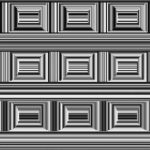Forum Options

Home Forums SYSTEM Bit Error Rate# Bit Error Rate

How does the BER Analyser or even the Eye diagram Analyser give the BER value? I get BER values as low as 10^-52 even when I am transmitting a sequence length of 128 bits with 64 samples per bit at a data rate of 10E6 bps. Is this correct ? What could be the reason ?

### Responses (1):

• #50548How does the BER Analyser or even the Eye diagram Analyser give the BER value? I get BER values as low as 10^-52 even when I am transmitting a sequence length of 128 bits with 64 samples per bit at a data rate of 10E6 bps. Is this correct ? What could be the reason ?

• #50593